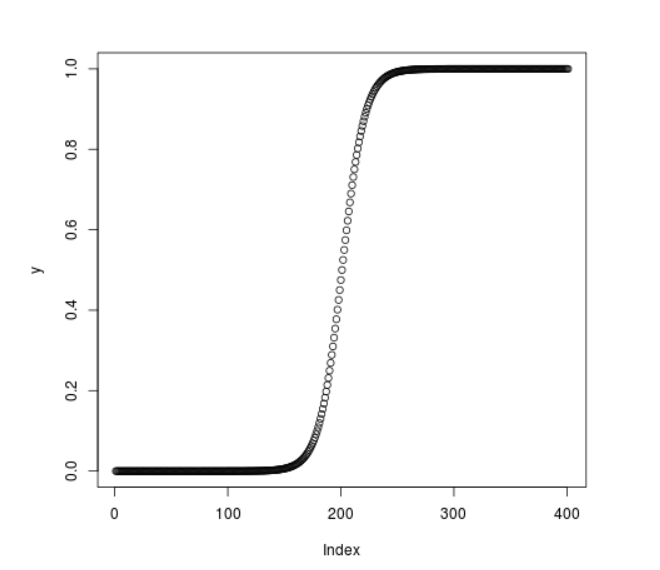# Compute Cumulative Logistic Density in R Programming – plogis() Function

• Last Updated : 25 Jun, 2020

`plogis()` function in R Language is used to compute logistic cumulative density of the distribution. It also creates a plot of the density of the logistic cumulative distribution.

Syntax: plogis(vec)

Parameters:
vec: Vector of x-values for density

Example 1:

 `# R program to calculate ``# cumulative logistic density`` ` `# Create a vector of x-values``x <``-` `seq(``-``1``, ``1``, by ``=` `0.1``)`` ` `# Calling the plogis() function``y <``-` `plogis(x)``y`

Output:

```  0.2689414 0.2890505 0.3100255 0.3318122 0.3543437 0.3775407 0.4013123
 0.4255575 0.4501660 0.4750208 0.5000000 0.5249792 0.5498340 0.5744425
 0.5986877 0.6224593 0.6456563 0.6681878 0.6899745 0.7109495 0.7310586
```

Example 2:

 `# R program to calculate ``# cumulative logistic density`` ` `# Create a vector of x-values``x <``-` `seq(``-``20``, ``20``, by ``=` `0.1``)`` ` `# Calling the plogis() function``y <``-` `plogis(x)`` ` `# Plot the graph``plot(y)`

Output:My Personal Notes arrow_drop_up